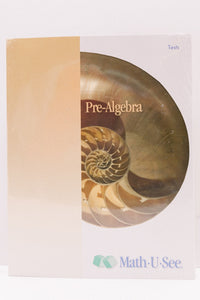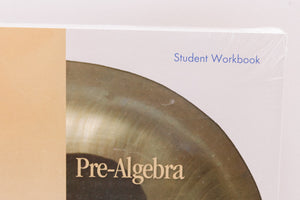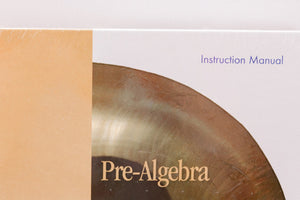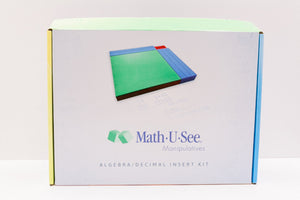# PreAlgebra Level Up Set Math U See (New)

Regular price
Sold out
Sale price
\$99.00

Major Concepts and Skills Include:

• Fluently adding, subtracting, multiplying, and dividing positive and negative numbers
• Understanding and simplifying exponents
• Using properties of operations, order of operations, and absolute value
• Expanding understanding of place value
• Identifying the least common multiple and greatest common factor

• Using the Pythagorean theorem to identify triangle attributes
• Transforming temperatures between Celsius and Fahrenheit
• Understanding and computing surface area and volume
• Adding and subtracting time using both 12-hour and military notation